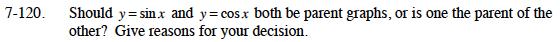Home > CCA2 > Chapter 7 > Lesson 7.2.1 > Problem7-120

7-120.

Should y = sin x and y = cos x both be parent graphs, or is one the parent of the other? Give reasons for your decision. Homework Help ✎Notice that the sine curve could be shifted horizontally to match the cosine curve, or vice-versa.
What is the horizontal shift?

$\text{Since } y = \text{cos }x \text{ is the same as }y = \text{sin }\left(x+\frac{\pi}{2}\right),\text{ only one of these needs to be the parent graph.}$